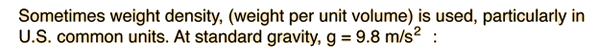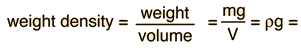# Density

Density is defined as mass per unit volume.Data can be entered into any of the boxes below. Specifying any two of the quantities determines the third. After you have entered values for two, click on the text representing to third to calculate its value.

gm/cm3 = gm / cm3
kg/m3 = kg / m3

The density of water is 1 gram/cm3 since water was used as an early mass standard, one gram being informally defined as the mass of 1 cm3 of water.N/m3= lb/ft3

### Table of densities.

Index

 HyperPhysics***** Mechanics R Nave
Go Back